# Find out what is the function SEARCHV in Excel and examples to stop wasting time searching for data

Are you looking for information about the function SEARCH and examples of use? do you need to automate your information management and want to find useful Excel formulas? you have come to the right place!

The SEARCH function in Excel is undoubtedly one of the most important intermediate formulas you need to learn in order to develop your accounting projects in the most optimal and efficient way. VLOOKUP is, in fact, the most used function of the Search and Reference group in Excel.

According to Julieta Santarelli, CPA with a master’s degree in Management & Analytics, who teaches the Basic Excel for Beginners course, “the search functions in Excel are a great help, since they allow you to locate data automatically within a spreadsheet”.

So, if you want to master the SEARCH function in Excel like an expert, keep reading. In this post, we will show you how the SEARCH function works in Excel, examples of how to use it and some tips to get the most out of it and maximize your productivity.

want to get the math straight, let’s get started!

## what is the SEARCH function in Excel for?

The first thing is to understand how the function SEARCHV in Excel works: the V refers to vertical searches, which clearly respond to the Cartesian language of Excel’s nature: to find points of coincidence between rows and columns.

In short, as quoted by the Excel Total portal, specialized in Excel, the SEARCHV function in Excel searches vertically (from top to bottom) “a certain value within a column of data and returns a value from a different column, but from the same row as the value found”.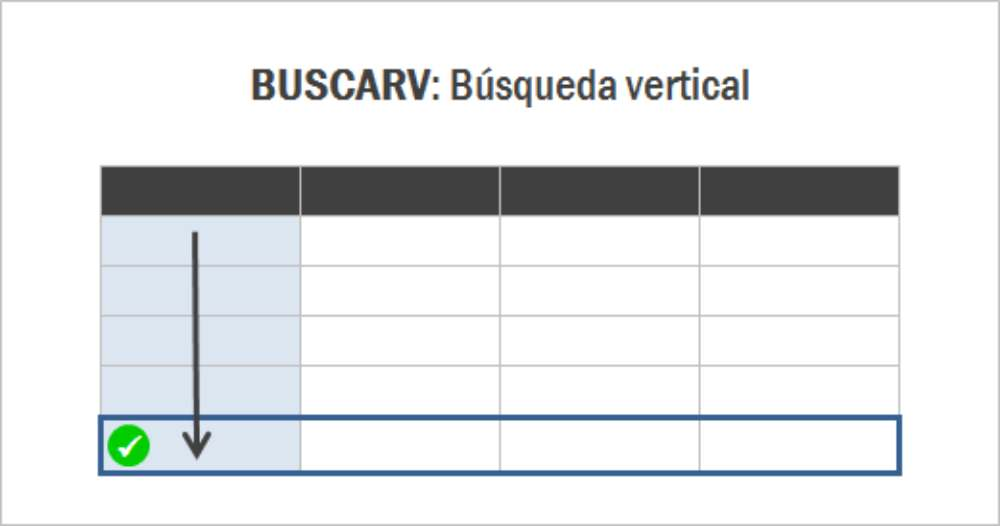Source: Excel Total

So, if you tell the SEARCHV function in Excel what data you want to search for, it will crawl cell by cell in a column until it finds the one that matches the row you set.

In short, the VLOOKUP function in Excel is used to find items in a table or a range by row. For example, you can search for the price of a product by name.

A curious fact is that when Excel 2010 was released, the function VLOOKUP changed its name to CONSULTAV, which generated a lot of confusion among users, so Microsoft decided to reverse the name change.

## what is the syntax of the SEARCH function in Excel?

According to Microsoft Support, in its simplest form, the SEARCHV function in Excel indicates the following:

=SEARV(What you want to search for; where you want to search; the column number in the range containing the value to return; returns an Exact Match (TRUE) or Approximate Match (FALSE)).

It is then understood that the syntax of the function SEARCHV in Excel has four arguments: =SEARCHV(Argument1,Argument2,Argument3,Argument4).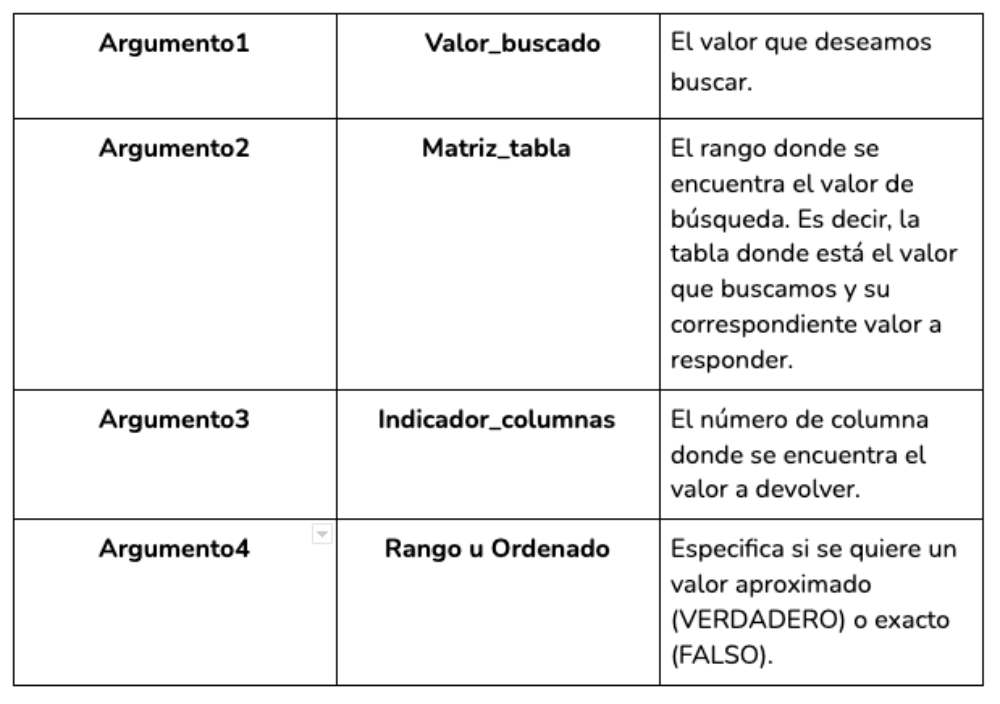Source: Excel

With the following image, you can better understand the syntax and how the function SEARCHV is composed.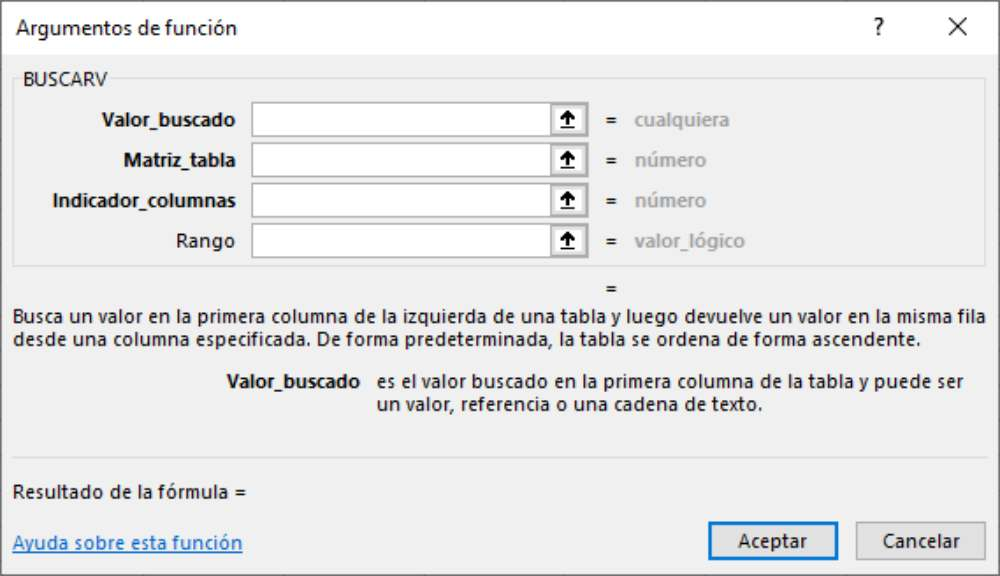Source: Excel Total

If up to this point you still don’t understand, don’t despair. let’s go with the examples so you can learn how to use the function SEARCHV in Excel. But first, we leave you some recommendations for you to prepare your data well before starting .

The data must have a tabular format; in rows and columns.
The column where the search will be performed should always be located to the left of the column that will be returned as a result. In other words, the column with the search data should be the first column of the data.
If an approximate search is performed, then the column to be searched should be sorted in ascending order.

## Examples of the SEARCHV function in Excel

Now let’s go through some step-by-step examples of the SEARCHV function in Excel.

### 1. Example of SEARCH for sales

In this first example to learn how to use the SEARCH function in Excel we will use a list of sales with the following data: Food, Category, Price, Units and Sales. In this way, we detail the name of the food (Tomato, Celery, Oranges or Carrots), what type of food it is (fruit or vegetable) and its respective price per unit.

On the other hand, in a second table, we have what Julieta Santarelli calls a “dictionary table“, which contains all the fixed characteristics of the products.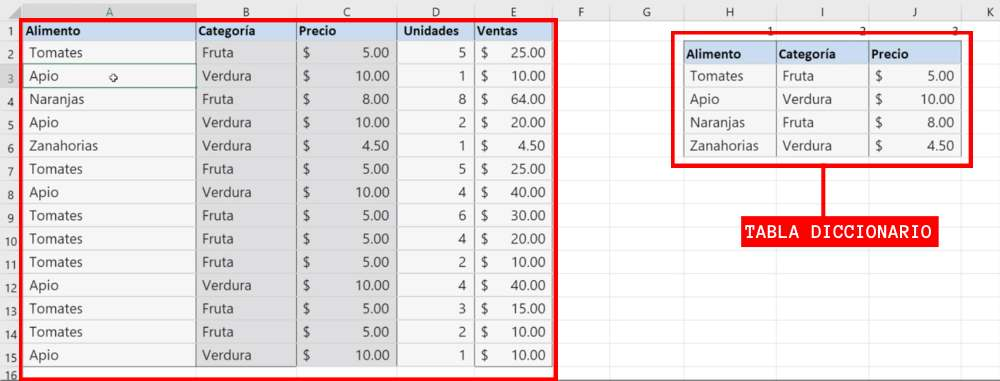For this example, using the SEARCHV function in Excel, let’s look up which category is the food ‘Tomato’.

Let’s identify each of the arguments:

Argument1: the cell with the name of the food item: A2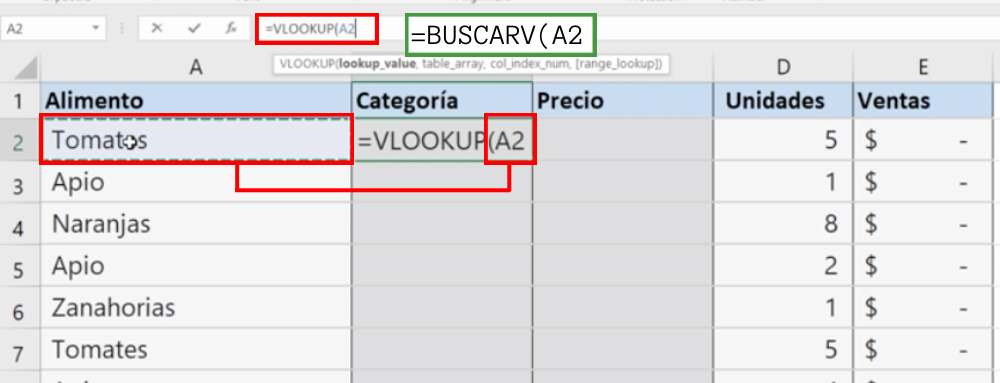Argument2: the ‘dictionary table’. For this example, it would be from cell H2 to J6. That is: H2:J6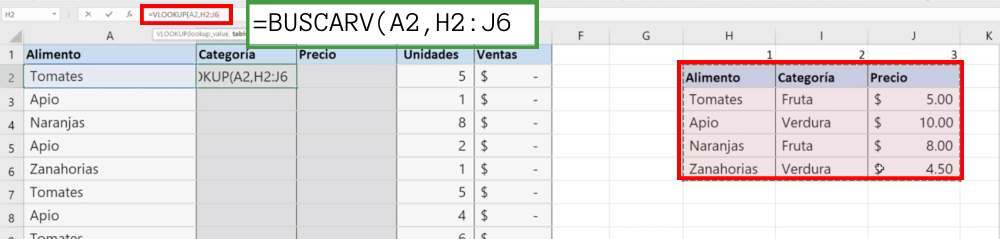argument3: the column number where the value to return is located. In this case, it is 2, since it is the one that defines the category of the product we want to query.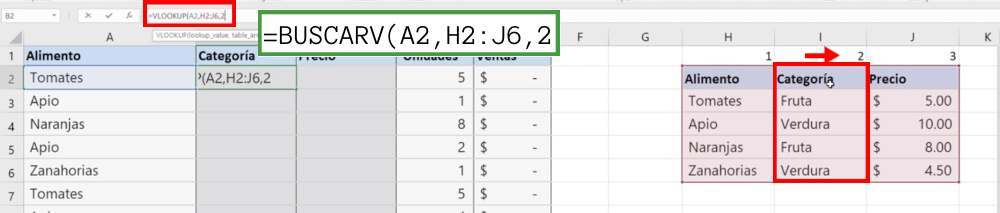Argument4: For our example we will put FALSE, because we want it to be exact.With all the elements together, the formula to quote in the search cell would look like this: =FINDV(A2,H2:J6,2,FALSE)

When closing parentheses, the value that the SEARCHV formula has to return is ‘Fruit’.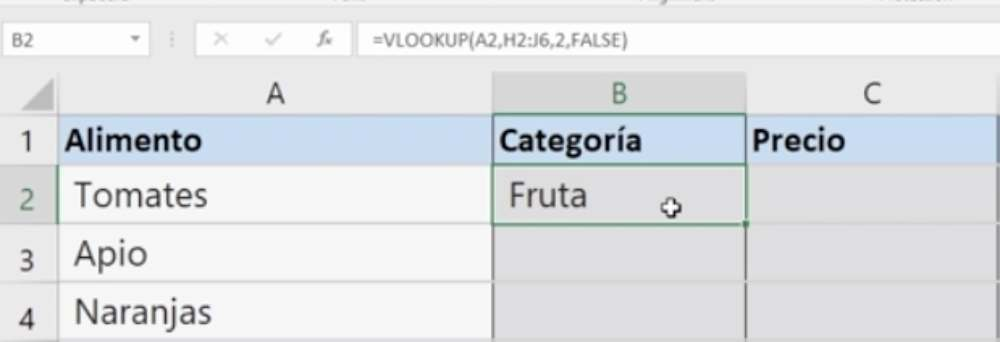### 2. Example of BUSCARV for Marketing

In the following example, published by the website BUSCARV, the intention is to search for certain information in a listing with YouTube videos.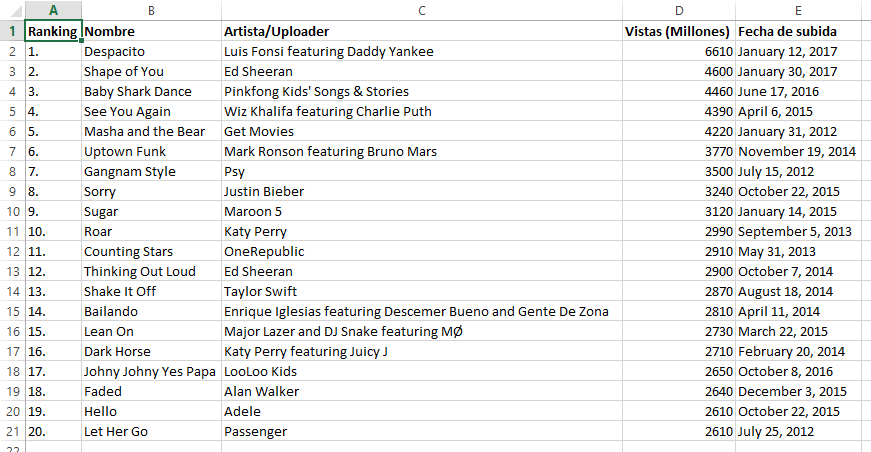Source: BUSCARV

The first application of BUSCARV in this example is to find the number of views that the video number 10 in the ranking has. The formula to use would be:

=SEARCHV(“10.”;A1:E21;4;FALSE)

Search_value: “10.” allows you to indicate the value to search for. It is important to enclose it in quotation marks. You can also put “10*” (the asterisk replaces any character) or the reference to a cell.
Array_search_in: A1:E21 allows us to indicate the range of cells in which the searched values and the values to query are located. However, if we are going to add more data, we can also put “A:E”. If all the rows of columns A, B, C, D and E are specified as a matrix, the formula will not change, even if more records are added to the end of the list.
Columns indicator allows to set that the millions of hits are 4 (coincidentally coincides with the column number).
[Ordered]: false allows an exact match, although in this case it would not be strictly necessary.

In this case, the result of the SEARCHV function is 2990.

### 3. Example of BUSCARV for product inventory

A customer requests a Rolling Stones CD, so we have to look for the shelf where it is located according to the following CD inventory. The inventory provides information about artist, album, media, store and shelf.

The first step is to enter the SEARCHV formula that will allow us to search for this information. To do so, we enter:

=SEARCHV(H2;A2:E9;5;FALSE)

Inside the brackets, first, we select the box where we place the information to search. Then, we place the range of data where we are going to look for the value.

After that, the column where the value to be found is located and the match to be achieved. and that’s it! You already have the formula SEARCHV to get the necessary information.

can you imagine what the result will be? of course! It is 18.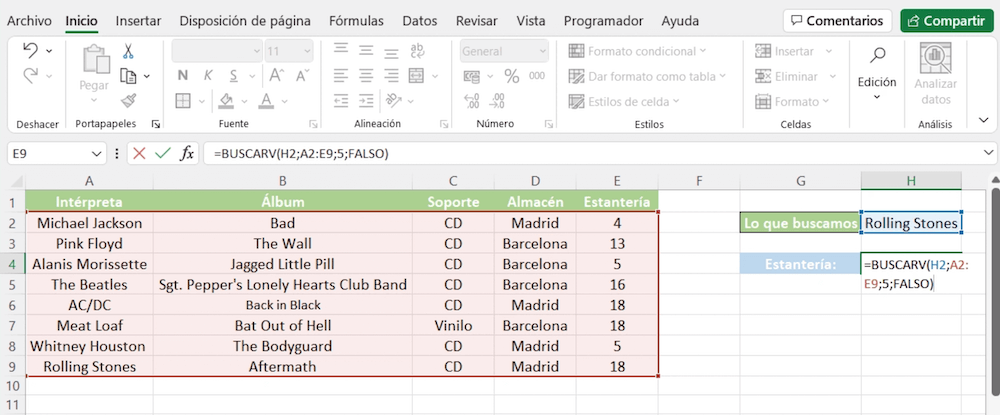Source: Citizen2zero

### 4. Example of BUSCARV for work teams

In the following table we detail a list of employees with their respective personal data. With the function SEARCHV, we are going to create a formula that allows us to obtain the name of a person just by providing the ID.

In cell G1 we are going to place the number of the employee whose name we want to know. For this example, we will use the ID 7746.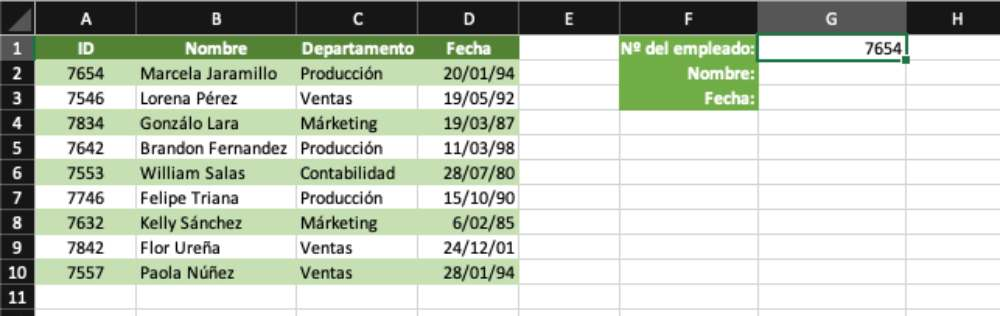Source: Excel

We are going to put the formula “=BUSCARV(“ in cell G2. For this example, we will use the <insert function> button , which is another way to insert the function SEARCHV, although not necessary. This time we will do it just to finish getting a complete overview of how the SEARCH function works.

But if you want, you can do the exercise just by typing the formula directly in the box: =FIND(G1;A2:D10;2;FALSE)

let’s continue!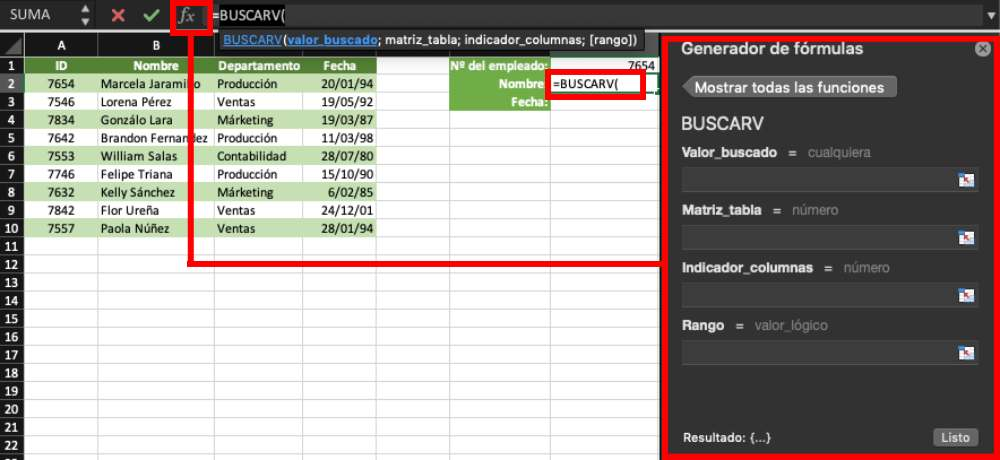Source: Excel

Argument1 is the Search_Value. In this case, it would be cell G1, where the ID of the employee whose name we want to know.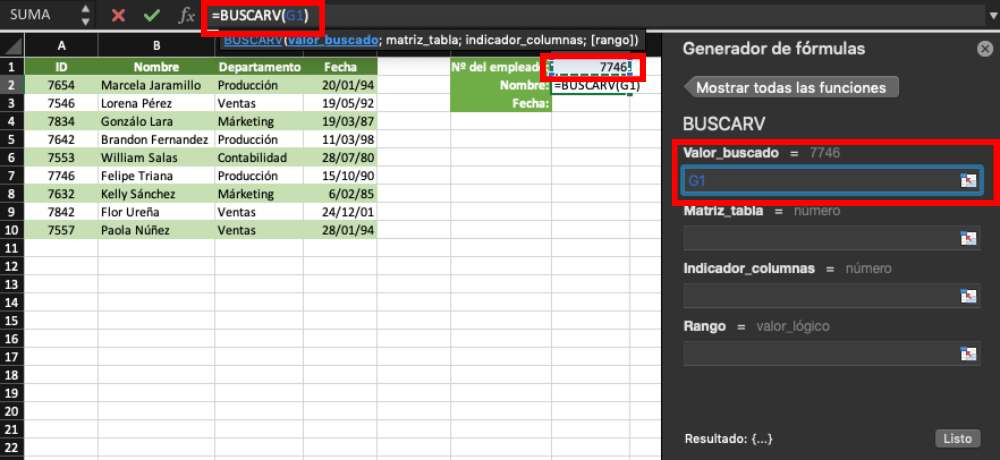Source: Excel

Argument2 is the Table_Matrix, that is, the range of cells where the data is located. Note that the table has a header (from cells A1 to D1), which you should omit; the range to insert in the formula should be A2:D10.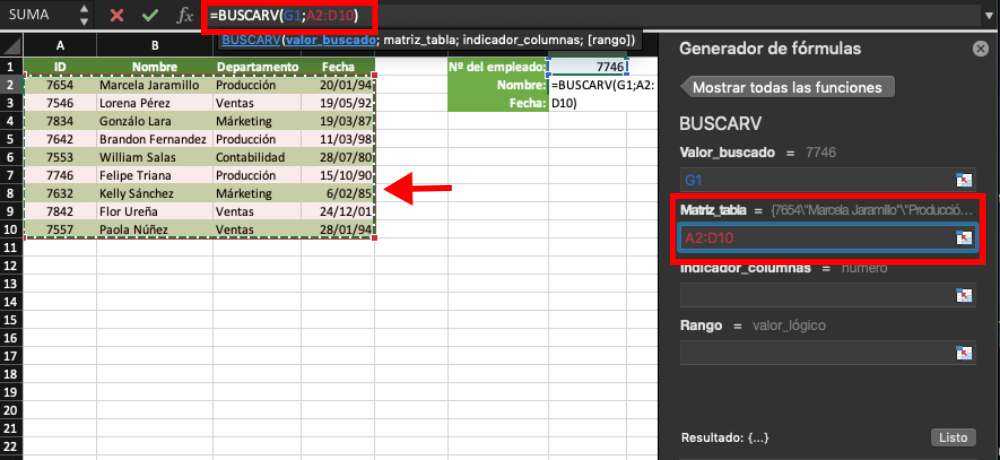Source: Excel

Argument3 is the Columns_indicator, to indicate to the function SEARCHV in Excel the column that we are interested in obtaining, which in this case would be the number 2, where the names of the employees are located.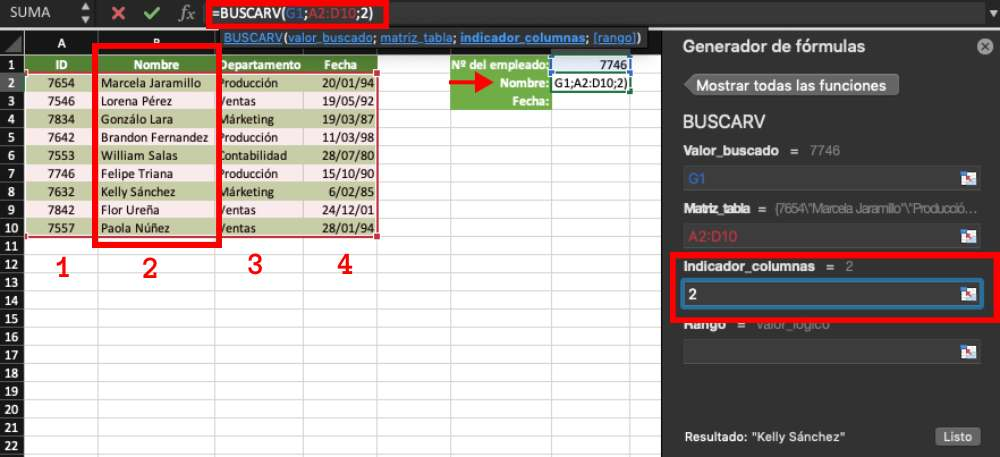Source: Excel

Argument4 is the Range or Sorted and should be FALSE, to indicate to the function that I must find an exact match of the searched value.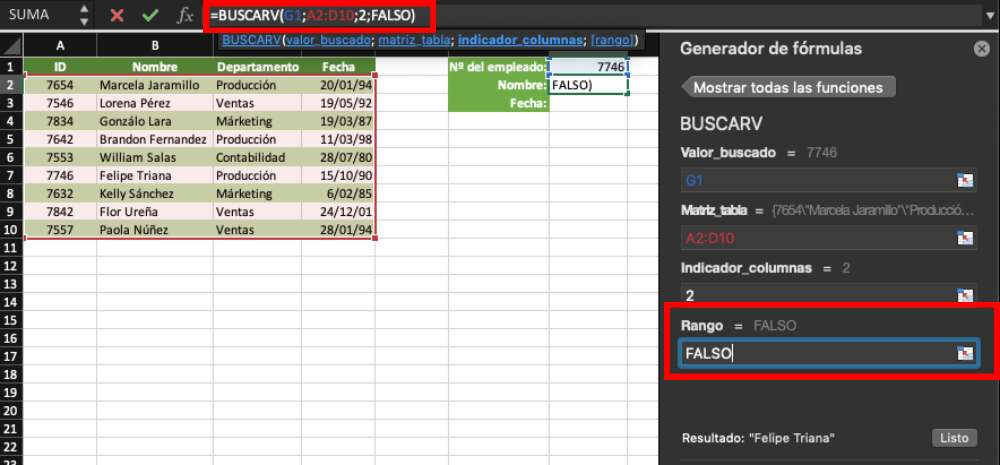Source: Excel

When you press the <OK> or <DONE> button, the function SEARCHV should have found the name “Felipe Triana”, to which the ID 7746 corresponds.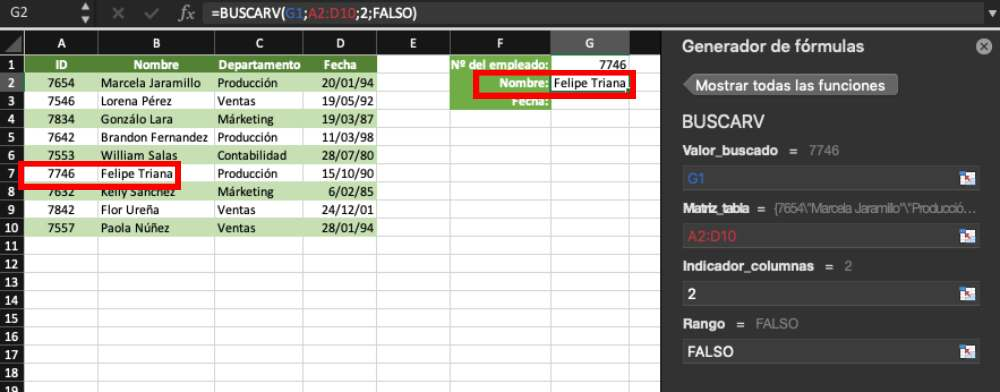Source: Excel

### 5. Example of BUSCARV to search for people data

Continuing with the data from the previous example, we apply the function SEARCHV in Excel again, but this time in cell G3, to find the date of birth of the employee in question: Felipe Triana.

Thus, three of the four arguments would be exactly the same:

Argument1: G1
Argument2: A2;D10
Argument4: FALSE

However, in the Argument3/Indicado_columns we must change the column number in which we will perform the search with the function BUSCARV, which in this case would be the number 4, where the dates are located; thus: =BUSCARV(G1;A2:D10;4;FALSE).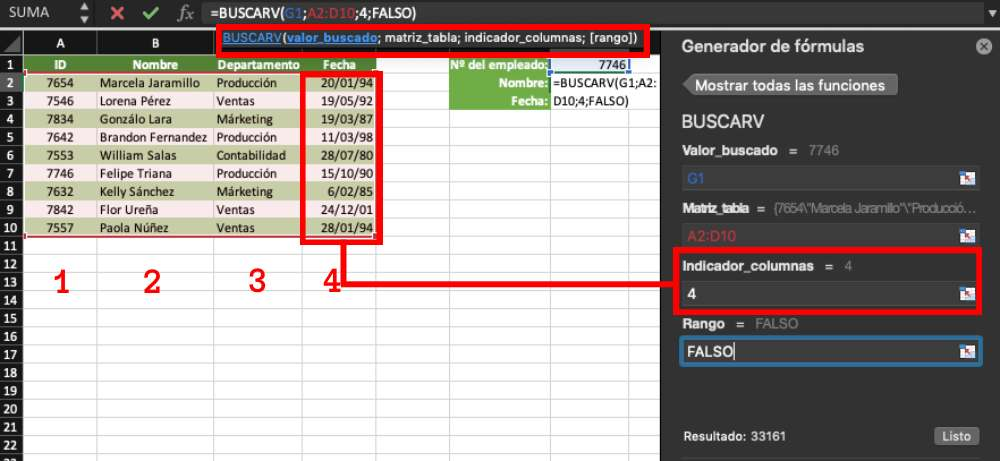Source: Excel

The result should be the date 10/15/90.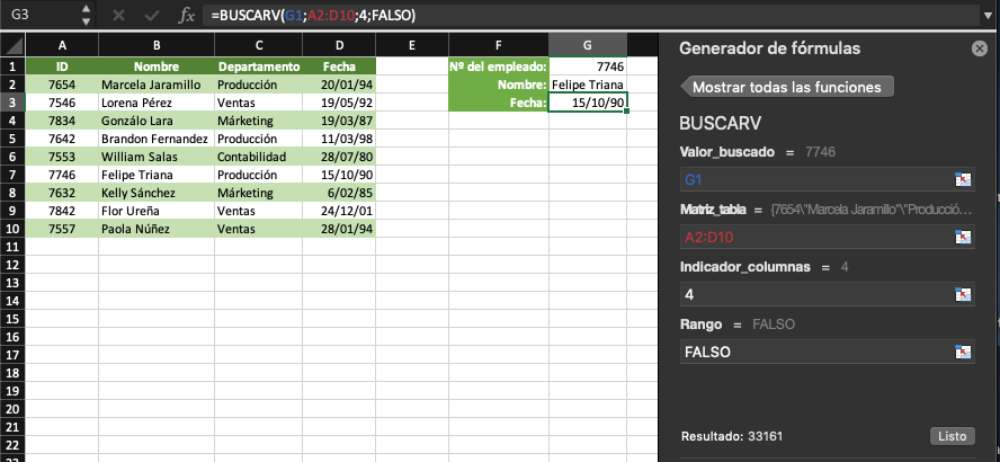Source: Excel

Finally, thanks to the function SEARCHV in Excel we have an automatic table designed to find employee data, because if you enter in the box G1 the ID of another person, his name and date of birth will appear immediately.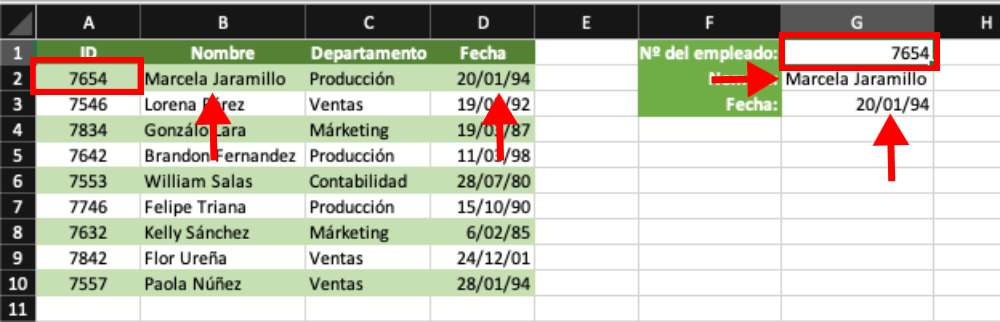Source: Excel

In this sense, if you enter an ID that does not exist in the data range or in the ‘dictionary table’, the formulas will return an error, as we will see in the following image.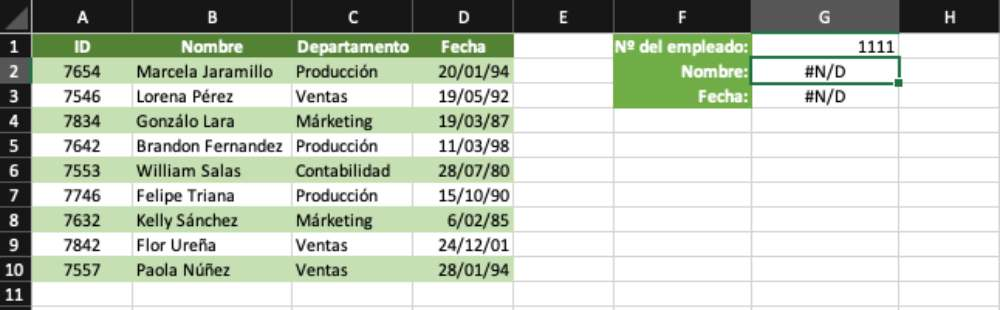Source: Excel

## Exercise to practice the SEARCHV function

To put into practice everything you learned about the SEARCH function in Excel with the examples, how about adding the Department box to the table above? Here we leave you the data: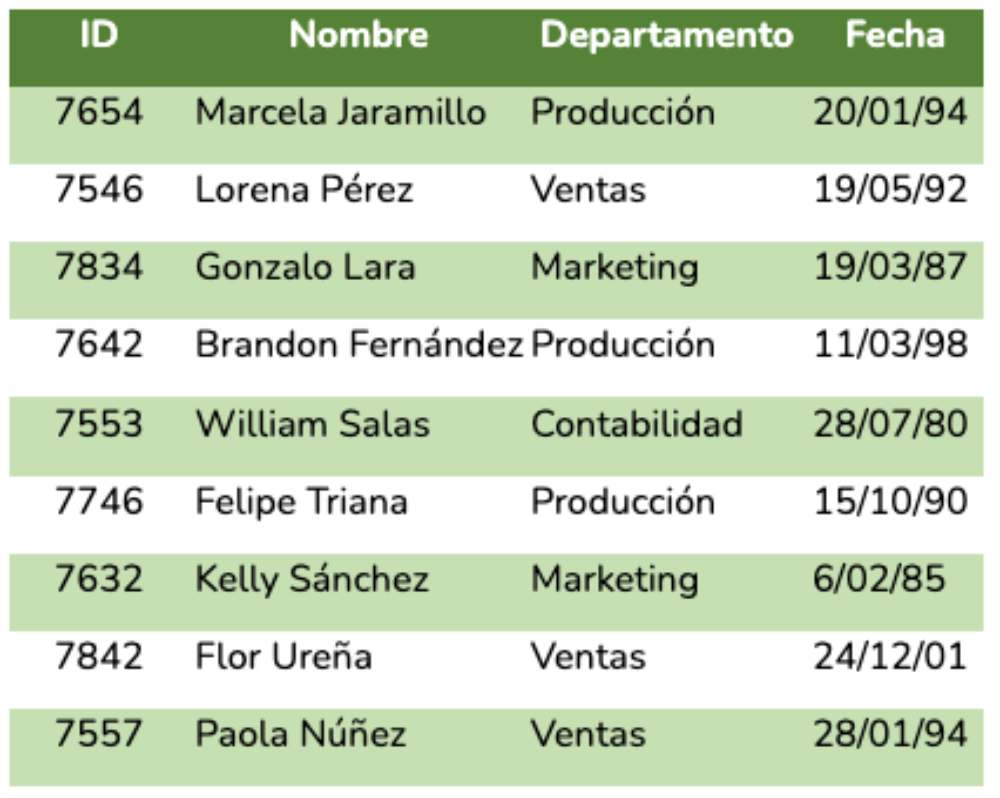For this exercise, you will only need to change, again, the Argument3; this time, for column number 3, which contains the data of the departments to which each employee belongs. With Excel’s function SEARCHV, this formula would be: =SEARCHV(G1;A2:D10;3;FALSE).

## Common errors of the function SEARCHV in Excel

have you ever tried the SEARCH function in Excel and the examples we shared with you above, but you got some errors? This is common even for Excel experts .

That’s why we share with you some of the most common SEARCH in Excel errors and their possible solutions, according to Microsoft’s support website. take note!

#N/A: if sorted is TRUE and the value in search_value is smaller than the smallest value in the first column of the search_array_in, the result value will be #N/A. If ordered is FALSE, the error value #N/A in SEARCHV indicates that the exact number was not found.

#REF!: If column_indicator is greater than the number of columns in search_array_in, the returned value will be a #REF! error value.

#VALUE!: If array_search_in is less than 1, the returned value will be an error #REF! error value.

#NAME: The #NAME? error value in SEARCHV usually means that the formula is missing quotation marks. To search for a person’s name, you must make sure that the name is enclosed in quotes in the formula. For example, type the name as “Lopez” in =SEARV(“Lopez”;B2:E7;2;FALSE).

#SPILL!: The #SPILL! error in SEARCHV means that the formula relies on implicit intersection for the search value and on the use of an entire column as a reference. For example, =BUSCARV(A:A;A:C;2;FALSE). To solve the problem you can delimit the search reference with the @ operator as follows: =BUSCARV(@A:A;A:C;2;FALSE). Alternatively, you can use the traditional FINDV method and refer to a single cell instead of a whole column: =FALSEARV(A2;A:C;2;FALSE).

we’ve reached the end! Tell us, did you learn how to use the SEARCH function in Excel?
see you soon!